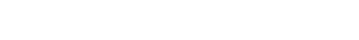# Superelevation in Highways -Analysis and DesignReading time: 1 minute

## What is Superelevation in Highway Engineering?

Superelevation is the transverse slope provided to counteract the effect of centrifugal force and reduce the tendency of vehicle to overturn and to skid laterally outwards by raising the pavement outer edge with respect to inner edge. superelevation is represented by “ e ”.Superelevation in Highway Engineering

## Analysis of superelevationLet us say Design speed = V m/s Radius = R m

### Various forces acting on the vehicle:Where e = rate of superelevation in % f = lateral friction factor = 0.15 V = velocity of vehicle in m/s g = acceleration due to gravity = 9.81 m/s2 R = radius of circular curve in meters.### Limits for maximum superelevation:

According to IRC the Maximum Superelevation in Areas Which Are Bound by Snow fall – 7% Which are not bounded by Snowfall – 10%

### Limits for minimum superelevation

Minimum superelevation = camber or cross slope Camber: Slope provide in the transverse direction to drain off rain water quickly is known as Camber or Cross slope. This will also prevents slipping and skidding of vehicles.

## IRC Recommendations for Camber

 Type of pavement Light rainfall intensity Heavy rainfall intensity C.C pavements and thick bituminous pavements 1 in 60 1 in 50 Thin Bituminous Pavements 1 in 50 1 in 40 W.B.M and Gravel Pavements 1 in 40 1 in 33 Earthen Pavements 1 in 33 1 in25

## Design of superelevation

There are four steps involved in the design of superelevation. And they are,

### Step 1:

Calculate the superelevation necessary for 75% design speed and assume No lateral friction is developedIf e value is less than emax = 0.07, provide calculated e value. Otherwise proceed to next step

### Step 2:

When ecal > emax Provide e = emax = 0.07 in this step and go to next step.

### Step 3:

From the above step we have the value of e. so, check for lateral friction factor is applied in this step for the known value of e.If fcal < fmax (0.15) Then e = 0.07 is safe. But if fcal > 0.15 Then restrict the values to f = 0.15, e = 0.07 And go to last step.

### Step 4:

In this step we will find out the value of restricted speed. Let V = VaIf Va > V, then e = 0.07, f= 0.15 If Va < V , then also e = 0.07, f = 0.15 but, speed restriction board is provided which consists the value of Va As shown in figure below.